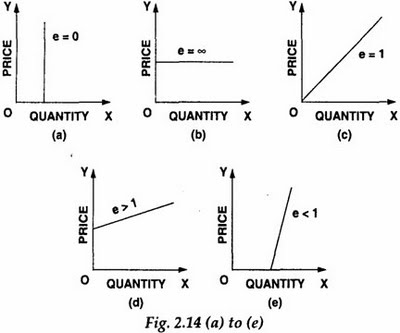# Types of Elasticity in Economics

2
396

## Types of Elasticity in Economics

ELASTICITY
Elasticity is a term widely used in economics to denote the “responsiveness of one variable to changes in another.” In proper words, it is the relative response of one variable to changes in another variable.
The phrase “relative response” is best interpreted as the percentage change.

TYPES OF ELASTICITY
There are four major types of elasticity:
• Price Elasticity of Demand
• Price Elasticity of Supply
• Income Elasticity of Demand
• Cross-Price Elasticity of Demand

Price Elasticity of Demand:
Price elasticity of demand is the percentage change in quantity demanded with respect to the percentage change in price.
Price elasticity of demand can be illustrated by the following formula:
PЄd =  Percentage change in Quantity Demanded
Percentage change in Price
Where Є = Epsilon; universal notation for elasticity.
If, for example, a 20% increase in the price of a product causes a 10% fall in the Quantity demanded,
the price elasticity of demand will be:
PЄd = – – 10%  = – 0.5
20%

Price Elasticity of Supply:
Price elasticity of supply is the percentage change in quantity supplied with respect to the percentage change in price.
Price elasticity of supply can be illustrated by the following formula:
PЄs =  Percentage change in Quantity Supplied
Percentage change in Price
If a 15% rise in the price of a product causes a 15% rise in the quantity supplied, the price elasticity of
Supply will be:
PЄs = 15 %  = 1
15 %
Introduction to Economics –ECO401 VU

Income Elasticity of Demand:
Income elasticity of demand is the percentage change in quantity demanded with respect to the
percentage change in income of the consumer.
Income elasticity of demand can be illustrated by the following formula:
YЄd =  Percentage change in Quantity Demanded
Percentage change in Income
If a 2% rise in the consumer’s incomes causes an 8% rise in product’s demand, then the income
elasticity of demand for the product will be:
YЄd = 8%  =4
2%

Cross-Price Elasticity of Demand:
Cross price elasticity of demand is the percentage change in quantity demanded of a specific good, with
respect to the percentage change in the price of another related good.

PbЄda  = Percentage change in Demand for good a
Percentage change in Price of good b
If, for example, the demand for butter rose by 2% when the price of margarine rose by 8%, then the cross price elasticity of demand of butter with respect to the price of margarine will be.

PbЄda = 2%  = 0.25
8%

If, on the other hand, the price of bread (a compliment) rose, the demand for butter would fall. If a 4% rise in the price of bread led to a 3% fall in the demand for butter, the cross-price elasticity of demand
for butter with respect to bread would be:
PbЄda = – 3% = – 0.75
4%

Virtual University